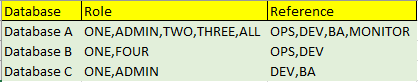# LINQ expression for Data Table agregation

Hello,

I have the next data table

Database Role Reference
Database A ONE OPS,DEV
Database B ONE OPS,DEV
Database C ONE DEV
Database A TWO BA
Database A THREE BA
Database B FOUR DEV
Database A ALL MONITOR

I want to group all the rows by “Database” and have all the “Roles” & “References” of the same datebase agregated in the same cell. The last two columns “Role” & “Reference” can be of Type String OR Type Array.

In the result, I want to obtain this sorted data tableCould you please help me with a LINQ Query to group the databases and select many roles and references ?

@SONYC
Welcome to the forum

Assign Activity
dtResult = yourOrigDTVar.Clone

Assign Activity
dtResult =

``````(From d in yourOrigDTVar.AsEnumerable
Group d by k=d("Database").toString.Trim into grp=Group
Let jr = String.Join(",",grp.Select(Function (x) x("Role")))
Let rr = String.Join(",",grp.Select(Function (x) x("Reference")))
Let ra = New Object(){k, jr, rr}
``````
1 Like

Thank you Peter!

I get the expected result except the thing that I want to remove the duplicate values.

I tryed this, but it’s taking only the value from the first line of the database group.

(From d in yourOrigDTVar.AsEnumerable
Group d by k=d(“Database”).toString.Trim into grp=Group
Let jr = String.Join(“,”,grp.Select(Function (x) x(“Role”)).First)
Let rr = String.Join(“,”,grp.Select(Function (x) x(“Reference”)).First)
Let ra = New Object(){k, jr, rr}

Hi,

We need to use Distinct method as the following, for example.

``````(From d In dt.AsEnumerable
Group d By k=d("Database").toString.Trim Into grp=Group
Let jr = String.Join(",",grp.Select(Function (x) x("Role")).Distinct)
Let rr = String.Join(",",grp.Select(Function (x) x("Reference")).Distinct)
Let ra = New Object(){k, jr, rr}
``````

Sample20221217-1aL.zip (2.9 KB)

Regards,

1 Like

Thank you Yoichi!

I just managed to use .Distinct method and I was about to post the solution.

Thank you both for your help!

1 Like

How could I ignor case while selecting distinct values ?

I might have the same reference in lower case and in upper case as well.

Hi,

How could I ignor case while selecting distinct values ?

``````(From d In dt.AsEnumerable
Group d By k=d("Database").toString.Trim Into grp=Group
Let jr = String.Join(",",String.Join(",",grp.Select(Function (x) x("Role").ToString)).Split(","c).Distinct(StringComparer.InvariantCultureIgnoreCase))
Let rr = String.Join(",",String.Join(",",grp.Select(Function (x) x("Reference").ToString)).Split(","c).Distinct(StringComparer.InvariantCultureIgnoreCase))
Let ra = New Object(){k, jr, rr}
``````

Regards,

Thank you again Yoichi!

The split was necessary as I still got the result with duplicate values in some cases where I had multiple references separated by “,” in the same cell.

This expression fits perfectly to my needs.

Kind regards.

1 Like

This topic was automatically closed 3 days after the last reply. New replies are no longer allowed.